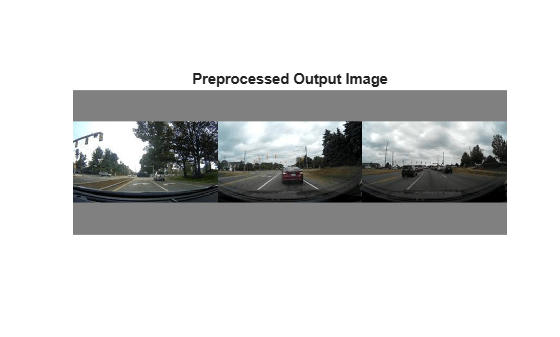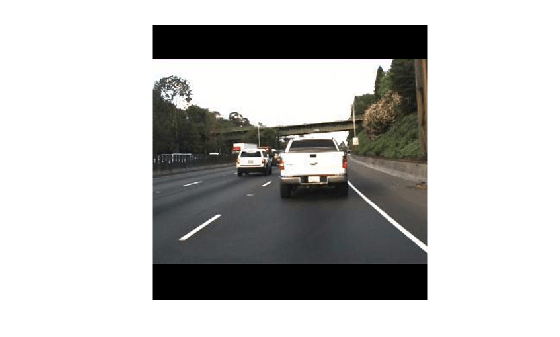# preprocess

Preprocess training and test images

## Syntax

``outputData = preprocess(detector,trainingData)``
``outputImg = preprocess(detector,img)``
``[___,scaleInfo] = preprocess(___)``

## Description

example

````outputData = preprocess(detector,trainingData)` preprocesses the training data `trainingData` before using it to train the YOLO v3 object detector. The training images and the corresponding bounding boxes are stored in the `trainingData`. The `preprocess` function performs these operations: Rescales the intensity values of the training images to the range [0, 1].Resizes the training images to one of the nearest network input sizes and updates the bounding box coordinate values for accurate training. The function preserves the original aspect ratio of the training data. ```

example

````outputImg = preprocess(detector,img)` preprocesses the test images `img` for object detection using a YOLO v3 object detector. The `preprocess` function performs these operations: Rescales the intensity values of the test images to the range [0, 1].Resizes the test images to one of the nearest network input sizes and preserves the original aspect ratio of each test image. ```
````[___,scaleInfo] = preprocess(___)` returns information on the scale factor applied for image resizing, in addition to any combination of arguments from previous syntaxes. NoteThis function requires the Computer Vision Toolbox™ Model for YOLO v3 Object Detection. You can install the Computer Vision Toolbox Model for YOLO v3 Object Detection from Add-On Explorer. For more information about installing add-ons, see Get and Manage Add-Ons. To run this function, you will require the Deep Learning Toolbox™. ```

## Examples

collapse all

Load a pretrained YOLO v3 object detector.

`detector = yolov3ObjectDetector('tiny-yolov3-coco');`

Load the training dataset into the workspace. The training data is a cell array that contains the images, bounding box values, and the class labels.

`load('trainingData.mat','trainingData');`

Resize the training images to the nearest network input size and rescale the intensity values by using the `preprocess` function.

`outputData = preprocess(detector,trainingData);`

Display the output image and the scale information used for resizing the image.

```outputImg = outputData{1,1}; figure imshow(outputImg)```Display the input and the preprocessed values.

`bboxIn = cell2table(trainingData,'VariableNames',{'Images','Bounding Boxes','Labels'})`
```bboxIn=3×3 table Images Bounding Boxes Labels _________________ ________________________ ___________ {224×399×3 uint8} 220 136 35 28 {'vehicle'} {224×399×3 uint8} 175 126 61 45 {'vehicle'} {224×399×3 uint8} 108 120 45 33 {'vehicle'} ```
`bboxOut = cell2table(outputData,'VariableNames',{'Images','Bounding Boxes','Labels'})`
```bboxOut=3×3 table Images Bounding Boxes Labels __________________ ________________________ ___________ {416×416×3 single} 229 232 36 29 {'vehicle'} {416×416×3 single} 182 222 64 46 {'vehicle'} {416×416×3 single} 112 215 47 35 {'vehicle'} ```

Load a pretrained YOLO v3 object detector.

`detector = yolov3ObjectDetector('tiny-yolov3-coco');`

`I = imread('highway.png');`

Resize the test image to the network input size and rescale the intensity values by using the `preprocess` function.

`[outputImg,scaleInfo] = preprocess(detector,I);`

Display the output image and the scale information used for resizing the image.

```figure imshow(outputImg)````disp(scaleInfo)`
``` PreprocessedImageSize: [416 416] ScaleX: 0.7692 ScaleY: 0.5769 ```

## Input Arguments

collapse all

YOLO v3 object detector, specified as a `yolov3ObjectDetector` object.

Training data for YOLO v3 object detector, specified as a N-by-3 cell array that contains the images, bounding boxes, and the class labels. Each row is of the form [images bounding boxes labels]. N is the number of output layers in the network. The bounding boxes must be stored as a K-by-4 matrix of the form [x y width height]. K is the number of object classes.

Test images, specified as a numeric array of size M-by-N-by-C or M-by-N-by-C-by-T. M is the number of rows, N is the number of columns, and C is the number of color channels. The value of C is `1` for grayscale images and `3` for RGB color images. T is the number of test images in the array.

Data Types: `single` | `double` | `int8` | `int16` | `int32` | `int64` | `uint8` | `uint16` | `uint32` | `uint64`

## Output Arguments

collapse all

Preprocessed training data, returned as a N-by-3 cell array.

Data Types: `cell`

Preprocessed test images, returned as a numeric array of size P-by-Q-by-C or P-by-Q-by-C-by-T. P and Q are the number of rows and columns, respectively, in the preprocessed image.

Data Types: `single`

Information about the scale factor for resizing the input images, returned as a structure with fields `PreprocessedImageSize`, `ScaleX`, and `ScaleY`.

• `PreprocessedImageSize` — Size of the output resized image.

• `ScaleX` — Scale factor for resizing an image in the X-direction (along the rows).

• `ScaleY` — Scale factor for resizing an image in the Y-direction (along the columns).

Data Types: `struct`